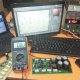Breaking News
Home / Electronics Help Care

## Electronics Help CareRamen Bairagee

## ampere meter voltmeter connection, electronics

Ampere meter voltmeter connection, electronics: This is a voltage and ampere measurement meter. we can measure voltage and ampere. this meter can run 5-25 voltage. we can see it has 2 types of wire. two-socket has in this meter. 1st socket has 3 wire. Red, black and yellow. The red …

## Voltage controller circuit with ampere using IRF540N? electronics

Voltage controller circuit with ampere using IRF540N: This is a Voltage controller circuit with ampere using IRF540N. IRF540N. This transistor can take 33Amperes and  100Voltage. 0.040 Ohm, N-channel Power MOSFET. This is only DC voltage Regulator. we can control 0 voltage to 100 voltage. But here in this video, I …

## 4 1 amplifier schematic diagram, TDA7388

4.1 amplifier schematic diagram: This is a 4.1 amplifier schematic diagram, this diagram we can use as a car amplifier, or also we can use in the home, this is home theater amplifier also. here in this circuit diagram can make 200 watts. par speaker 50 watts. 50+50+50+50=200 watts. TDA7388 …

## how to make bass circuit diagram? low pass filter circuit diagram, 100% working, electronics

how to make bass circuit diagram? low pass filter circuit diagram, 100% working, electronics: This is a low pass filter circuit diagram. in this article, we can learn how to make the bass circuit diagram? low pass filter circuit diagram. normally our amplifier is not made the only bass. if …

## How to make inverter 12v dc to 220v ac? making circuit diagram? making transformer? electronics

How to make inverter 12v dc to 220v ac? making a circuit diagram? making a transformer? electronics: this is an inverter making circuit diagram, here we are using only two MOSFET because it’s only 100 watts inverter. we use the ic ka3525a for the oscillation. in this circuit only can …

## How to make copper transformer? rewinding copper transformer, electronics

How to make a copper transformer? rewinding copper transformer: Normally we don’t find a copper transformer to the electronics market. For this, we have to reuse the old copper transformer. We can find old copper transformer in the old tv, in the computer power supply. in many where. but we …

## How to make transistor amplifier using 4 transostor? electronics

How to make a transistor amplifier using 2 transistors, electronics: We are going to make an amplifier circuit diagram using 4 transistors. 2sa1943 and 2sc5200. it’s 200 Watts circuit diagram. 2sa1943 and 2sc5200 both transistor can take 1.3 amperes. We know that voltage X amperes = Watts.        …

## How to make transistor amplifier? using 2 transostor, electronics

How to make a transistor amplifier using 2 transistors? electronics: This is 2 transistors amplifier circuit diagram. it’s 2sc5200 and 2sa1943 transistor circuit diagram. it’s 100 watts amplifier circuit diagram. this transistor can take a maximum of 1.3 amperes. so 1.3X2=2.6 amperes. if we use 40 voltage and 2.5 or …